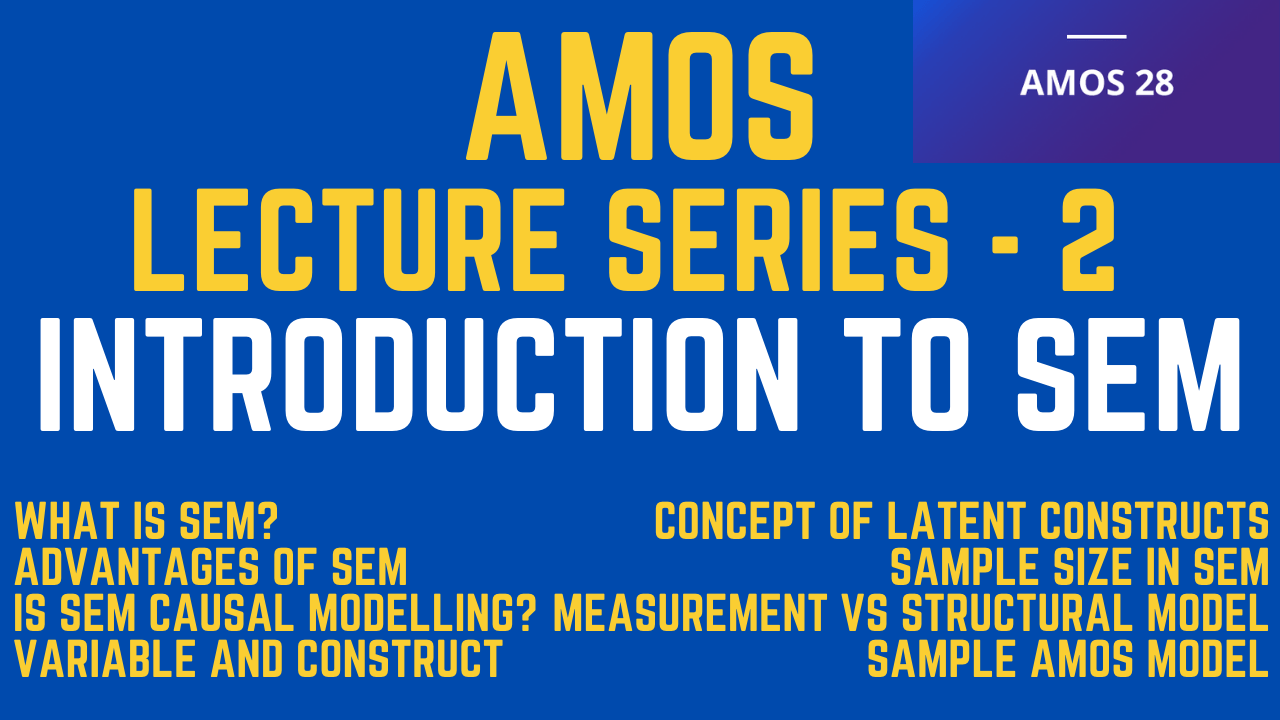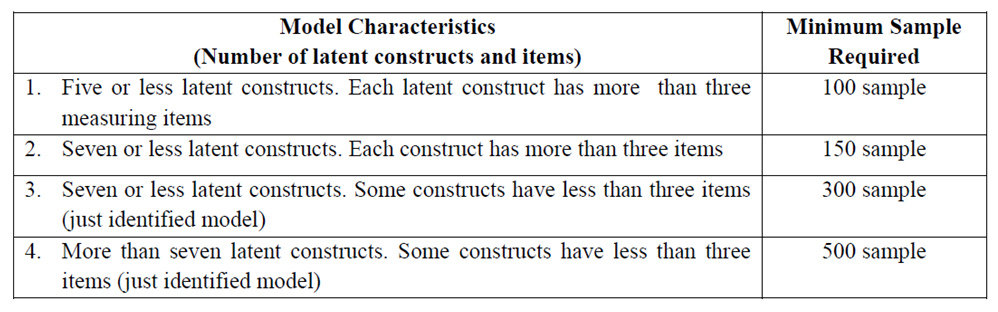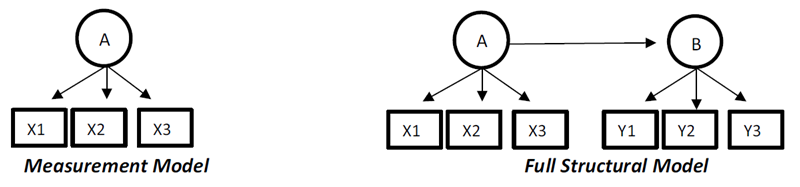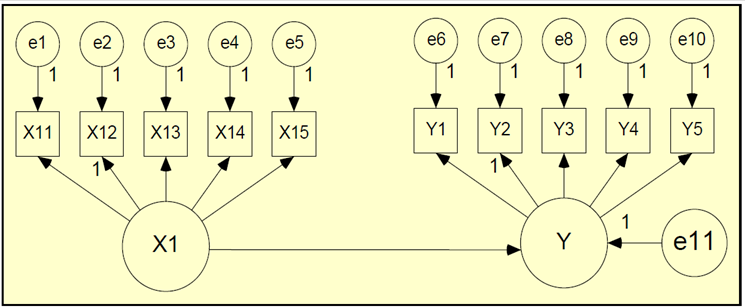# SPSS AMOS Series - A Detailed Introduction of SEM and Its Concepts

## Introduction to Structural Equation Modelling

The session will introduce some of the most basic and important concepts in Structural Equation Modelling (SEM). The session will cover

• What is SEM?
• Is SEM Causal Modelling?
• Variables and Construct
• Concept of Latent Constructs
• Sample size in SEM
• Measurement vs Structural Model
• A Sample AMOS Model### IBM SPSS AMOS - Lecture Series - 2

Lecture 2 of the IBM SPSS AMOS Series. The lecture is a detailed introduction to different concepts that are critical to learning Structural Equation Modelling using AMOS

## What is SEM?

• Structural equation modeling, or SEM, is a statistical method that examines the relationships among numerous variables in a simultaneous way.
• SEM is not considered a single procedure but rather a family of related statistical techniques.
• This family of analysis techniques examines the measurement properties of a variable along with the interrelationships between variables and is often seen as a combination of regression and factor analysis.
• The use of SEM will often take a confirmatory approach in which the researcher has proposed a “model” of relationships between variables of interest and examines whether the observed data will provide evidence of directionality and significance of the relationships.
• SEM is very similar to multiple regression but is much more robust and has greater flexibility in the analysis. SEM allows you to model multiple independent and dependent variables, error terms, interactions, and correlations.
• Using a SEM model will also let you denote which independent variables will influence dependent variables, and subsequently, let dependent variables be independent variables in other relationships.
• Structural equation modeling is fundamentally built around the idea of modeling or drawing a model that represents relationships between variables.
• This model will use symbols to represent variables, relationships between variables, and even error in your model.
• SEM begins with a theory where the researcher intends to test the relationship among constructs of interest in the study. The relationships are modeled into a theoretical framework represented by a schematic diagram.
• The schematic diagram presents the hypotheses of interest to be tested in the study.
• The constructs of interest involved are measured using a set of items in a questionnaire.
• The measurement scale for each item should be either interval or ratio.

The main advantages of structural equation modeling compared to other techniques are

• It lets you analyze the influence of predictor variables on numerous dependent variables simultaneously.
• It allows you to account for measurement error and even addresses error in predicting relationships, and
• It is capable of testing an entire model instead of just focusing on individual relationships. This is in direct contrast to similar techniques like regression that can test only one dependent variable at a time, does not account for measurement error, and focuses on singular relationships instead of the collective whole.

## Is SEM Causal Modelling?

• You will often hear SEM referred to as a causal modeling approach. SEM does not determine causation between two variables.
• This is a contradiction that is used quite often with SEM. As stated earlier, SEM uses a covariance matrix as its input, so you are essentially looking at correlations between variables to determine how one influences the other, but it will not determine causation.
• Just because two things are highly correlated does not mean one causes the other.
• SEM is a great technique to determine how variables influence one another, but to determine causation you would really need to use an experimental design.
• While the majority of SEM research has been performed with non-experimental data, SEM is more than capable of analyzing experimental data.

## Variable vs Construct

• Throughout the series of videos, the viewers would find the term variable and construct are used interchangeably.
• A variable is meant for the directly measured score such as age, exam score, income etc.,
• While the construct is meant for an indirectly measured score such as Job Satisfaction, Perceived Usefulness, and Loyalty Intentions.
• In fact, the construct is a hypothetical concept of something, or the respondents’ perception concerning certain issue.
• A construct is measured through a set of items in a questionnaire.

## The Concept of Latent Constructs in Research

• In science and social science researches, most of the times the researchers are dealing with latent constructs.
• As has been said earlier, these constructs are measured using a set of items in a questionnaire. Since the Ordinary Least Squares (OLS) procedures could not entertain latent constructs, the researchers need to employ SEM for the analysis.
• Using SEM, the researcher could model the relationship among these constructs together with their respective items in the model and analyze them simultaneously.
• In this case, at least two measurement models involved – one for independent construct and the other one is for dependent construct.
• The theorized link between measurement model for independent construct and measurement model for dependent construct is called a structural model.
• Thus, instead of modeling the OLS regression and analyze using ANOVA, the researcher is working with the Structure Equation Modeling (SEM) and analyzed using software’s like AMOS.

## The Minimum Sample Size in SEM

• There are endless debates in the literatures as to how many respondents should be obtained in order to employ SEM.
• However, there is no clear-cut answers to it since every research differs (among other things) in term of the population characteristics, and the number of constructs employed in a model.
• Hair et al. (2010), offer the following suggestion for minimum sample size depending on the model complexity and basic measurement model characteristics.## Measurement vs Structural Model

• The measurement model in SEM is where the researcher is going to assess the validity of the indicators for each construct. After showing the validity of the measurement model, the researcher can proceed to the structural model.
• The structural model is concerned with the influence and significance between constructs. The term “full structural model” means that the measurement and structural relationships of each construct are included in the model testing.
• Parameters—the term “parameter” indicates the size and nature of the relationship between two objects in a model. Parameters can be fixed to a constant or can be estimated freely from the data. A parameter estimate will take place on the measurement level with indicators and error terms as well as on the structural level between constructs.## Sample AMOS Model• Note: X1 and Y are latent constructs. In Amos syntax, latent constructs are represented by the ellipses. The latent construct X1 is measured using items X11 to X15, while latent construct Y is measured using items Y1 to Y5. The measured items are represented by rectangles in the model. Sometimes (in literature) the measured items are called latent indicators.
• Key: X1 = Exogenous construct, while X11 to X15 is a set of 5 items to measure latent construct X1
• In the Amos diagram, e1 to e5 are errors in measurement for items X11 to X15
• Y = Endogenous construct, while Y1 to Y5 is a set of 5 items to measure latent construct Y
• In the Amos diagram, e6 to e10 are errors in measurement for items Y1 to Y5

## References

• Collier, J. E. (2020). Applied structural equation modeling using AMOS: Basic to advanced techniques. Routledge.
• Awang, Z. (2015). A Handbook on SEM (2nd Edition). Malaysia